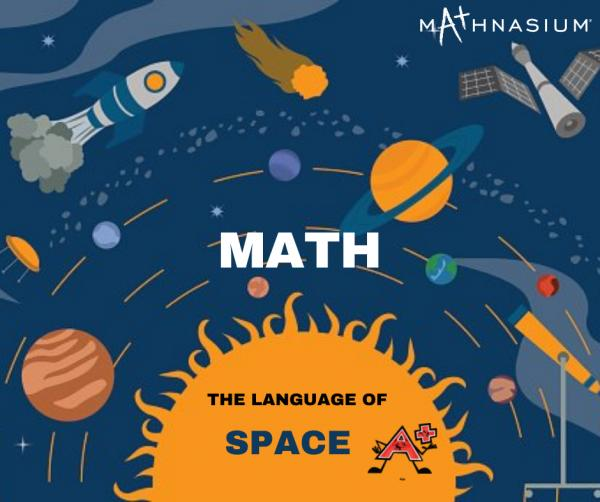* indicates a required field• phone number format invalid• email format invalidProblems detected, please review the form.
Privacy - Terms

# News from Mathnasium of South Beaverton

### Math Matters for Space Travel

Jan 5, 2022… 5 … 4 … 3 … 2 … 1 … and liftoff!

How does NASA get a satellite to orbit the Earth? What math powers rockets? How does math help us explore space?

Well, there are numerous ways how Math is used in Space Travel. Mathematics is the foundation of contemporary science and engineering. Let us take a quick and simple look at how these complex operations are done.

How To Calculate Required Fuel For a Rocket:

Isn't it amazing how scientists calculate the amount of fuel required to take the space shuttle to its destination? At liftoff, technicians know the two Solid Rocket Boosters consume 11,000 pounds of fuel per second. (That's two million times the rate at which fuel is burned by the average family car!) One of the most useful measures of a rocket's efficiency, however, is specific impulse. Specific impulse (Isp) is a measure of the mass that can be lifted by a given fuel system for each pound of fuel consumed per second of time. These values are all calculated with math to make sure the right amount of fuel is prepared based on the needs of the spacecraft and the voyage.

Uses of Calculus:

Calculus is a branch of Mathematics that helps us understand changes between values related by a function. Everything from designing rocket nozzles (heat distribution and fuel flow) to calculating launch, cruise, and return trajectories (many-body gravity, frictional forces, and integration of thrust vs. changing mass) to designing instruments, tools, modules, and space-suits (materials strength, wear characteristics, heat and air distribution, forces between objects) all use calculus. Calculus is how we keep our astronauts, spacecrafts, and space missions safe and successful.

Use of Coordinate System:

All of us have used GPS on the phone or in the car. GPS identifies the latitude and longitude to figure out your exact location, in order to give you the best directions to your destination. GPS in space is unfortunately not that simple.  Instead, the GPS operates in 3 dimensions using the coordinates X, Y, and Z in space to locate a spacecraft. Math is required to figure out where one is pointed, where it is going, and the best path of travel.

Finding & Calculating the Distance:

How do you calculate the distance between different planets, stars, and other space objects? Scientists send radio waves from Earth towards a galactic body in order to figure out the distance. Once they know the time it takes to reach the target, and the radio waves' speed, they can calculate the distance with math formulas.

Calculating the routes:

Mathematics plays an important role in space flight. It gives us a way both to predict what should happen in the future and ways to measure what is happening in the present to adapt to it.  When creating space-faring vehicles, designers use math to calculate distance, speed, velocity, and safety. Mathematics is the guide, instruction manual, and road map for optimal space travel.

In short, math is required for everything in space travel!